# Asset Pricing: Finite State Models¶

## Contents¶

“A little knowledge of geometric series goes a long way” – Robert E. Lucas, Jr.

“Asset pricing is all about covariances” – Lars Peter Hansen

In addition to what’s in Anaconda, this lecture will need the following libraries:

In :
!pip install quantecon


## Overview¶

An asset is a claim on one or more future payoffs.

The spot price of an asset depends primarily on

• the anticipated dynamics for the stream of income accruing to the owners
• attitudes to risk
• rates of time preference

In this lecture, we consider some standard pricing models and dividend stream specifications.

We study how prices and dividend-price ratios respond in these different scenarios.

We also look at creating and pricing derivative assets by repackaging income streams.

Key tools for the lecture are

• formulas for predicting future values of functions of a Markov state
• a formula for predicting the discounted sum of future values of a Markov state

Let’s start with some imports:

In :
import numpy as np
import matplotlib.pyplot as plt
%matplotlib inline
import quantecon as qe
from numpy.linalg import eigvals, solve

/home/ubuntu/anaconda3/lib/python3.7/site-packages/numba/np/ufunc/parallel.py:355: NumbaWarning: The TBB threading layer requires TBB version 2019.5 or later i.e., TBB_INTERFACE_VERSION >= 11005. Found TBB_INTERFACE_VERSION = 11004. The TBB threading layer is disabled.
warnings.warn(problem)


## Pricing Models¶

In what follows let $\{d_t\}_{t \geq 0}$ be a stream of dividends

• A time-$t$ cum-dividend asset is a claim to the stream $d_t, d_{t+1}, \ldots$.
• A time-$t$ ex-dividend asset is a claim to the stream $d_{t+1}, d_{t+2}, \ldots$.

Let’s look at some equations that we expect to hold for prices of assets under ex-dividend contracts (we will consider cum-dividend pricing in the exercises).

### Risk-Neutral Pricing¶

Our first scenario is risk-neutral pricing.

Let $\beta = 1/(1+\rho)$ be an intertemporal discount factor, where $\rho$ is the rate at which agents discount the future.

The basic risk-neutral asset pricing equation for pricing one unit of an ex-dividend asset is

$$p_t = \beta {\mathbb E}_t [d_{t+1} + p_{t+1}] \tag{1}$$

This is a simple “cost equals expected benefit” relationship.

Here ${\mathbb E}_t [y]$ denotes the best forecast of $y$, conditioned on information available at time $t$.

### Pricing with Random Discount Factor¶

What happens if for some reason traders discount payouts differently depending on the state of the world?

Michael Harrison and David Kreps [HK79] and Lars Peter Hansen and Scott Richard [HR87] showed that in quite general settings the price of an ex-dividend asset obeys

$$p_t = {\mathbb E}_t \left[ m_{t+1} ( d_{t+1} + p_{t+1} ) \right] \tag{2}$$

for some stochastic discount factor $m_{t+1}$.

The fixed discount factor $\beta$ in (1) has been replaced by the random variable $m_{t+1}$.

The way anticipated future payoffs are evaluated can now depend on various random outcomes.

One example of this idea is that assets that tend to have good payoffs in bad states of the world might be regarded as more valuable.

This is because they pay well when funds are more urgently wanted.

We give examples of how the stochastic discount factor has been modeled below.

### Asset Pricing and Covariances¶

Recall that, from the definition of a conditional covariance ${\rm cov}_t (x_{t+1}, y_{t+1})$, we have

$${\mathbb E}_t (x_{t+1} y_{t+1}) = {\rm cov}_t (x_{t+1}, y_{t+1}) + {\mathbb E}_t x_{t+1} {\mathbb E}_t y_{t+1} \tag{3}$$

If we apply this definition to the asset pricing equation (2) we obtain

$$p_t = {\mathbb E}_t m_{t+1} {\mathbb E}_t (d_{t+1} + p_{t+1}) + {\rm cov}_t (m_{t+1}, d_{t+1}+ p_{t+1}) \tag{4}$$

It is useful to regard equation (4) as a generalization of equation (1)

• In equation (1), the stochastic discount factor $m_{t+1} = \beta$, a constant.
• In equation (1), the covariance term ${\rm cov}_t (m_{t+1}, d_{t+1}+ p_{t+1})$ is zero because $m_{t+1} = \beta$.
• In equation (1), ${\mathbb E}_t m_{t+1}$ can be interpreted as the reciprocal of the one-period risk-free gross interest rate.
• When $m_{t+1}$ is covaries more negatively with the payout $p_{t+1} + d_{t+1}$, the price of the asset is lower.

Equation (4) asserts that the covariance of the stochastic discount factor with the one period payout $d_{t+1} + p_{t+1}$ is an important determinant of the price $p_t$.

We give examples of some models of stochastic discount factors that have been proposed later in this lecture and also in a later lecture.

### The Price-Dividend Ratio¶

Aside from prices, another quantity of interest is the price-dividend ratio $v_t := p_t / d_t$.

Let’s write down an expression that this ratio should satisfy.

We can divide both sides of (2) by $d_t$ to get

$$v_t = {\mathbb E}_t \left[ m_{t+1} \frac{d_{t+1}}{d_t} (1 + v_{t+1}) \right] \tag{5}$$

Below we’ll discuss the implication of this equation.

## Prices in the Risk-Neutral Case¶

What can we say about price dynamics on the basis of the models described above?

The answer to this question depends on

1. the process we specify for dividends
2. the stochastic discount factor and how it correlates with dividends

For now we’ll study the risk-neutral case in which the stochastic discount factor is constant.

We’ll focus on how the asset prices depends on the dividend process.

### Example 1: Constant Dividends¶

The simplest case is risk-neutral pricing in the face of a constant, non-random dividend stream $d_t = d > 0$.

Removing the expectation from (1) and iterating forward gives

\begin{aligned} p_t & = \beta (d + p_{t+1}) \\ & = \beta (d + \beta(d + p_{t+2})) \\ & \quad \vdots \\ & = \beta (d + \beta d + \beta^2 d + \cdots + \beta^{k-2} d + \beta^{k-1} p_{t+k}) \end{aligned}

Unless prices explode in the future, this sequence converges to

$$\bar p := \frac{\beta d}{1-\beta} \tag{6}$$

This price is the equilibrium price in the constant dividend case.

Indeed, simple algebra shows that setting $p_t = \bar p$ for all $t$ satisfies the equilibrium condition $p_t = \beta (d + p_{t+1})$.

### Example 2: Dividends with Deterministic Growth Paths¶

Consider a growing, non-random dividend process $d_{t+1} = g d_t$ where $0 < g \beta < 1$.

While prices are not usually constant when dividends grow over time, the price dividend-ratio might be.

If we guess this, substituting $v_t = v$ into (5) as well as our other assumptions, we get $v = \beta g (1 + v)$.

Since $\beta g < 1$, we have a unique positive solution:

$$v = \frac{\beta g}{1 - \beta g }$$

The price is then

$$p_t = \frac{\beta g}{1 - \beta g } d_t$$

If, in this example, we take $g = 1+\kappa$ and let $\rho := 1/\beta - 1$, then the price becomes

$$p_t = \frac{1 + \kappa}{ \rho - \kappa} d_t$$

This is called the Gordon formula.

### Example 3: Markov Growth, Risk-Neutral Pricing¶

Next, we consider a dividend process

$$d_{t+1} = g_{t+1} d_t \tag{7}$$

The stochastic growth factor $\{g_t\}$ is given by

$$g_t = g(X_t), \quad t = 1, 2, \ldots$$

where

1. $\{X_t\}$ is a finite Markov chain with state space $S$ and transition probabilities

$$P(x, y) := \mathbb P \{ X_{t+1} = y \,|\, X_t = x \} \qquad (x, y \in S)$$

2. $g$ is a given function on $S$ taking positive values

You can think of

• $S$ as $n$ possible “states of the world” and $X_t$ as the current state.
• $g$ as a function that maps a given state $X_t$ into a growth of dividends factor $g_t = g(X_t)$.
• $\ln g_t = \ln (d_{t+1} / d_t)$ is the growth rate of dividends.

(For a refresher on notation and theory for finite Markov chains see this lecture)

The next figure shows a simulation, where

• $\{X_t\}$ evolves as a discretized AR1 process produced using Tauchen’s method.
• $g_t = \exp(X_t)$, so that $\ln g_t = X_t$ is the growth rate.
In :
mc = qe.tauchen(0.96, 0.25, n=25)
sim_length = 80

x_series = mc.simulate(sim_length, init=np.median(mc.state_values))
g_series = np.exp(x_series)
d_series = np.cumprod(g_series) # Assumes d_0 = 1

series = [x_series, g_series, d_series, np.log(d_series)]
labels = ['$X_t$', '$g_t$', '$d_t$', r'$\log \, d_t$']

fig, axes = plt.subplots(2, 2, figsize=(12, 8))
for ax, s, label in zip(axes.flatten(), series, labels):
ax.plot(s, 'b-', lw=2, label=label)
ax.legend(loc='upper left', frameon=False)
plt.tight_layout()
plt.show()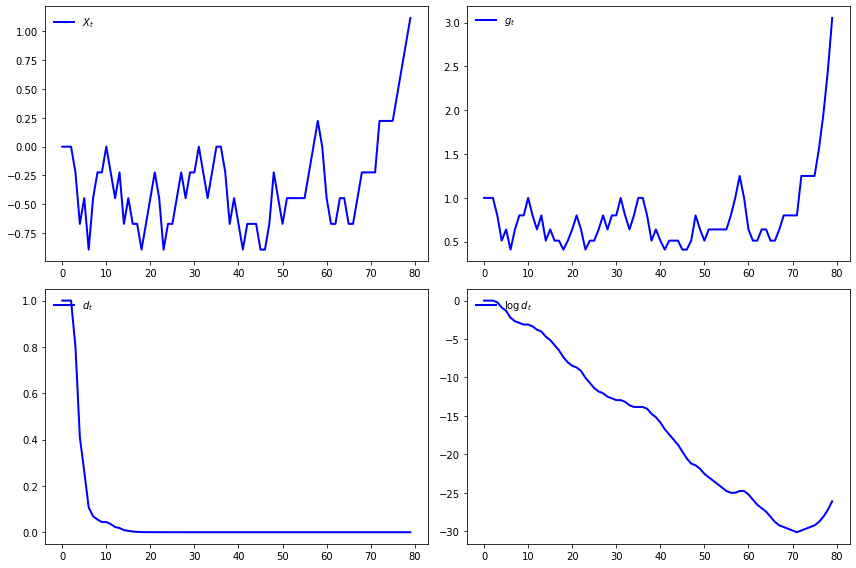#### Pricing¶

To obtain asset prices in this setting, let’s adapt our analysis from the case of deterministic growth.

In that case, we found that $v$ is constant.

This encourages us to guess that, in the current case, $v_t$ is constant given the state $X_t$.

In other words, we are looking for a fixed function $v$ such that the price-dividend ratio satisfies $v_t = v(X_t)$.

We can substitute this guess into (5) to get

$$v(X_t) = \beta {\mathbb E}_t [ g(X_{t+1}) (1 + v(X_{t+1})) ]$$

If we condition on $X_t = x$, this becomes

$$v(x) = \beta \sum_{y \in S} g(y) (1 + v(y)) P(x, y)$$

or

$$v(x) = \beta \sum_{y \in S} K(x, y) (1 + v(y)) \quad \text{where} \quad K(x, y) := g(y) P(x, y) \tag{8}$$

Suppose that there are $n$ possible states $x_1, \ldots, x_n$.

We can then think of (8) as $n$ stacked equations, one for each state, and write it in matrix form as

$$v = \beta K (\mathbb 1 + v) \tag{9}$$

Here

• $v$ is understood to be the column vector $(v(x_1), \ldots, v(x_n))'$.
• $K$ is the matrix $(K(x_i, x_j))_{1 \leq i, j \leq n}$.
• ${\mathbb 1}$ is a column vector of ones.

When does (9) have a unique solution?

From the Neumann series lemma and Gelfand’s formula, this will be the case if $\beta K$ has spectral radius strictly less than one.

In other words, we require that the eigenvalues of $K$ be strictly less than $\beta^{-1}$ in modulus.

The solution is then

$$v = (I - \beta K)^{-1} \beta K{\mathbb 1} \tag{10}$$

### Code¶

Let’s calculate and plot the price-dividend ratio at a set of parameters.

As before, we’ll generate $\{X_t\}$ as a discretized AR1 process and set $g_t = \exp(X_t)$.

Here’s the code, including a test of the spectral radius condition

In :
n = 25  # Size of state space
β = 0.9
mc = qe.tauchen(0.96, 0.02, n=n)

K = mc.P * np.exp(mc.state_values)

warning_message = "Spectral radius condition fails"
assert np.max(np.abs(eigvals(K))) < 1 / β,  warning_message

I = np.identity(n)
v = solve(I - β * K, β * K @ np.ones(n))

fig, ax = plt.subplots(figsize=(12, 8))
ax.plot(mc.state_values, v, 'g-o', lw=2, alpha=0.7, label='$v$')
ax.set_ylabel("price-dividend ratio")
ax.set_xlabel("state")
ax.legend(loc='upper left')
plt.show()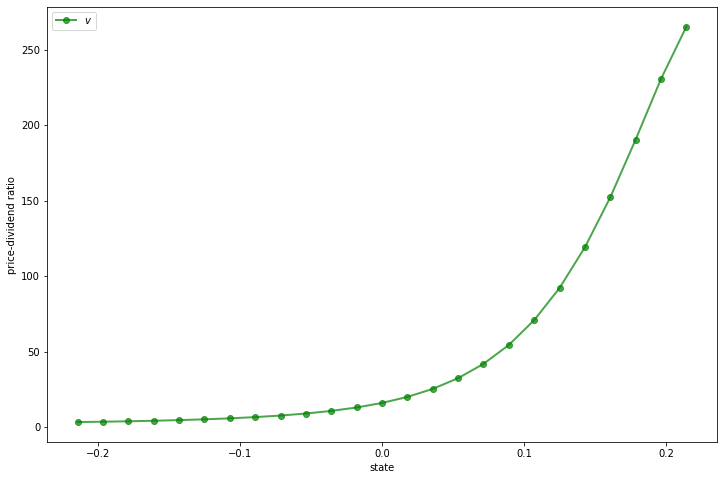Why does the price-dividend ratio increase with the state?

The reason is that this Markov process is positively correlated, so high current states suggest high future states.

Moreover, dividend growth is increasing in the state.

The anticipation of high future dividend growth leads to a high price-dividend ratio.

## Risk Aversion and Asset Prices¶

Now let’s turn to the case where agents are risk averse.

We’ll price several distinct assets, including

• An endowment stream
• A consol (a type of bond issued by the UK government in the 19th century)
• Call options on a consol

### Pricing a Lucas Tree¶

Let’s start with a version of the celebrated asset pricing model of Robert E. Lucas, Jr. [Luc78].

As in [Luc78], suppose that the stochastic discount factor takes the form

$$m_{t+1} = \beta \frac{u'(c_{t+1})}{u'(c_t)} \tag{11}$$

where $u$ is a concave utility function and $c_t$ is time $t$ consumption of a representative consumer.

(A derivation of this expression is given in a later lecture)

Assume the existence of an endowment that follows growth process (7).

The asset being priced is a claim on the endowment process.

Following [Luc78], suppose further that in equilibrium, consumption is equal to the endowment, so that $d_t = c_t$ for all $t$.

For utility, we’ll assume the constant relative risk aversion (CRRA) specification

$$u(c) = \frac{c^{1-\gamma}}{1 - \gamma} \ {\rm with} \ \gamma > 0 \tag{12}$$

When $\gamma =1$ we let $u(c) = \ln c$.

Inserting the CRRA specification into (11) and using $c_t = d_t$ gives

$$m_{t+1} = \beta \left(\frac{c_{t+1}}{c_t}\right)^{-\gamma} = \beta g_{t+1}^{-\gamma} \tag{13}$$

Substituting this into (5) gives the price-dividend ratio formula

$$v(X_t) = \beta {\mathbb E}_t \left[ g(X_{t+1})^{1-\gamma} (1 + v(X_{t+1}) ) \right]$$

Conditioning on $X_t = x$, we can write this as

$$v(x) = \beta \sum_{y \in S} g(y)^{1-\gamma} (1 + v(y) ) P(x, y)$$

If we let

$$J(x, y) := g(y)^{1-\gamma} P(x, y)$$

then we can rewrite in vector form as

$$v = \beta J ({\mathbb 1} + v )$$

Assuming that the spectral radius of $J$ is strictly less than $\beta^{-1}$, this equation has the unique solution

$$v = (I - \beta J)^{-1} \beta J {\mathbb 1} \tag{14}$$

We will define a function tree_price to solve for $v$ given parameters stored in the class AssetPriceModel

In :
class AssetPriceModel:
"""
A class that stores the primitives of the asset pricing model.

Parameters
----------
β : scalar, float
Discount factor
mc : MarkovChain
Contains the transition matrix and set of state values for the state
process
γ : scalar(float)
Coefficient of risk aversion
g : callable
The function mapping states to growth rates

"""
def __init__(self, β=0.96, mc=None, γ=2.0, g=np.exp):
self.β, self.γ = β, γ
self.g = g

# A default process for the Markov chain
if mc is None:
self.ρ = 0.9
self.σ = 0.02
self.mc = qe.tauchen(self.ρ, self.σ, n=25)
else:
self.mc = mc

self.n = self.mc.P.shape

def test_stability(self, Q):
"""
Stability test for a given matrix Q.
"""
sr = np.max(np.abs(eigvals(Q)))
if not sr < 1 / self.β:
msg = f"Spectral radius condition failed with radius = {sr}"
raise ValueError(msg)

def tree_price(ap):
"""
Computes the price-dividend ratio of the Lucas tree.

Parameters
----------
ap: AssetPriceModel
An instance of AssetPriceModel containing primitives

Returns
-------
v : array_like(float)
Lucas tree price-dividend ratio

"""
# Simplify names, set up matrices
β, γ, P, y = ap.β, ap.γ, ap.mc.P, ap.mc.state_values
J = P * ap.g(y)**(1 - γ)

# Make sure that a unique solution exists
ap.test_stability(J)

# Compute v
I = np.identity(ap.n)
Ones = np.ones(ap.n)
v = solve(I - β * J, β * J @ Ones)

return v


Here’s a plot of $v$ as a function of the state for several values of $\gamma$, with a positively correlated Markov process and $g(x) = \exp(x)$

In :
γs = [1.2, 1.4, 1.6, 1.8, 2.0]
ap = AssetPriceModel()
states = ap.mc.state_values

fig, ax = plt.subplots(figsize=(12, 8))

for γ in γs:
ap.γ = γ
v = tree_price(ap)
ax.plot(states, v, lw=2, alpha=0.6, label=rf"$\gamma = {γ}$")

ax.set_title('Price-divdend ratio as a function of the state')
ax.set_ylabel("price-dividend ratio")
ax.set_xlabel("state")
ax.legend(loc='upper right')
plt.show()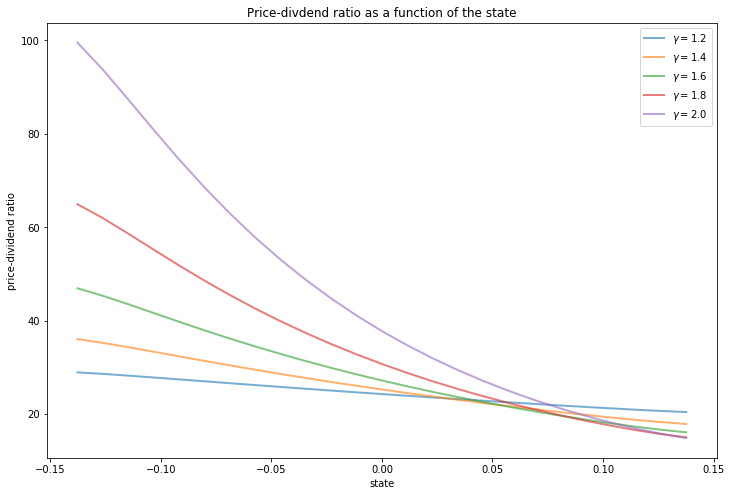Notice that $v$ is decreasing in each case.

This is because, with a positively correlated state process, higher states suggest higher future consumption growth.

In the stochastic discount factor (13), higher growth decreases the discount factor, lowering the weight placed on future returns.

#### Special Cases¶

In the special case $\gamma =1$, we have $J = P$.

Recalling that $P^i {\mathbb 1} = {\mathbb 1}$ for all $i$ and applying Neumann’s geometric series lemma, we are led to

$$v = \beta(I-\beta P)^{-1} {\mathbb 1} = \beta \sum_{i=0}^{\infty} \beta^i P^i {\mathbb 1} = \beta \frac{1}{1 - \beta} {\mathbb 1}$$

Thus, with log preferences, the price-dividend ratio for a Lucas tree is constant.

Alternatively, if $\gamma = 0$, then $J = K$ and we recover the risk-neutral solution (10).

This is as expected, since $\gamma = 0$ implies $u(c) = c$ (and hence agents are risk-neutral).

### A Risk-Free Consol¶

Consider the same pure exchange representative agent economy.

A risk-free consol promises to pay a constant amount $\zeta> 0$ each period.

Recycling notation, let $p_t$ now be the price of an ex-coupon claim to the consol.

An ex-coupon claim to the consol entitles the owner at the end of period $t$ to

• $\zeta$ in period $t+1$, plus
• the right to sell the claim for $p_{t+1}$ next period

The price satisfies (2) with $d_t = \zeta$, or

$$p_t = {\mathbb E}_t \left[ m_{t+1} ( \zeta + p_{t+1} ) \right]$$

We maintain the stochastic discount factor (13), so this becomes

$$p_t = {\mathbb E}_t \left[ \beta g_{t+1}^{-\gamma} ( \zeta + p_{t+1} ) \right] \tag{15}$$

Guessing a solution of the form $p_t = p(X_t)$ and conditioning on $X_t = x$, we get

$$p(x) = \beta \sum_{y \in S} g(y)^{-\gamma} (\zeta + p(y)) P(x, y)$$

Letting $M(x, y) = P(x, y) g(y)^{-\gamma}$ and rewriting in vector notation yields the solution

$$p = (I - \beta M)^{-1} \beta M \zeta {\mathbb 1} \tag{16}$$

The above is implemented in the function consol_price.

In :
def consol_price(ap, ζ):
"""
Computes price of a consol bond with payoff ζ

Parameters
----------
ap: AssetPriceModel
An instance of AssetPriceModel containing primitives

ζ : scalar(float)
Coupon of the console

Returns
-------
p : array_like(float)
Console bond prices

"""
# Simplify names, set up matrices
β, γ, P, y = ap.β, ap.γ, ap.mc.P, ap.mc.state_values
M = P * ap.g(y)**(- γ)

# Make sure that a unique solution exists
ap.test_stability(M)

# Compute price
I = np.identity(ap.n)
Ones = np.ones(ap.n)
p = solve(I - β * M, β * ζ * M @ Ones)

return p


### Pricing an Option to Purchase the Consol¶

Let’s now price options of varying maturities.

We’ll study an option that gives the owner the right to purchase a consol at a price $p_S$.

#### An Infinite Horizon Call Option¶

We want to price an infinite horizon option to purchase a consol at a price $p_S$.

The option entitles the owner at the beginning of a period either

1. to purchase the bond at price $p_S$ now, or
2. not to exercise the option to purchase the asset now but to retain the right to exercise it later

Thus, the owner either exercises the option now or chooses not to exercise and wait until next period.

This is termed an infinite-horizon call option with strike price $p_S$.

The owner of the option is entitled to purchase the consol at price $p_S$ at the beginning of any period, after the coupon has been paid to the previous owner of the bond.

The fundamentals of the economy are identical with the one above, including the stochastic discount factor and the process for consumption.

Let $w(X_t, p_S)$ be the value of the option when the time $t$ growth state is known to be $X_t$ but before the owner has decided whether to exercise the option at time $t$ (i.e., today).

Recalling that $p(X_t)$ is the value of the consol when the initial growth state is $X_t$, the value of the option satisfies

$$w(X_t, p_S) = \max \left\{ \beta \, {\mathbb E}_t \frac{u'(c_{t+1})}{u'(c_t)} w(X_{t+1}, p_S), \; p(X_t) - p_S \right\}$$

The first term on the right is the value of waiting, while the second is the value of exercising now.

We can also write this as

$$w(x, p_S) = \max \left\{ \beta \sum_{y \in S} P(x, y) g(y)^{-\gamma} w (y, p_S), \; p(x) - p_S \right\} \tag{17}$$

With $M(x, y) = P(x, y) g(y)^{-\gamma}$ and $w$ as the vector of values $(w(x_i), p_S)_{i = 1}^n$, we can express (17) as the nonlinear vector equation

$$w = \max \{ \beta M w, \; p - p_S {\mathbb 1} \} \tag{18}$$

To solve (18), form the operator $T$ mapping vector $w$ into vector $Tw$ via

$$T w = \max \{ \beta M w,\; p - p_S {\mathbb 1} \}$$

Start at some initial $w$ and iterate with $T$ to convergence .

We can find the solution with the following function call_option

In :
def call_option(ap, ζ, p_s, ϵ=1e-7):
"""
Computes price of a call option on a consol bond.

Parameters
----------
ap: AssetPriceModel
An instance of AssetPriceModel containing primitives

ζ : scalar(float)
Coupon of the console

p_s : scalar(float)
Strike price

ϵ : scalar(float), optional(default=1e-8)
Tolerance for infinite horizon problem

Returns
-------
w : array_like(float)
Infinite horizon call option prices

"""
# Simplify names, set up matrices
β, γ, P, y = ap.β, ap.γ, ap.mc.P, ap.mc.state_values
M = P * ap.g(y)**(- γ)

# Make sure that a unique consol price exists
ap.test_stability(M)

# Compute option price
p = consol_price(ap, ζ)
w = np.zeros(ap.n)
error = ϵ + 1
while error > ϵ:
# Maximize across columns
w_new = np.maximum(β * M @ w, p - p_s)
# Find maximal difference of each component and update
error = np.amax(np.abs(w - w_new))
w = w_new

return w


Here’s a plot of $w$ compared to the consol price when $P_S = 40$

In :
ap = AssetPriceModel(β=0.9)
ζ = 1.0
strike_price = 40

x = ap.mc.state_values
p = consol_price(ap, ζ)
w = call_option(ap, ζ, strike_price)

fig, ax = plt.subplots(figsize=(12, 8))
ax.plot(x, p, 'b-', lw=2, label='consol price')
ax.plot(x, w, 'g-', lw=2, label='value of call option')
ax.set_xlabel("state")
ax.legend(loc='upper right')
plt.show()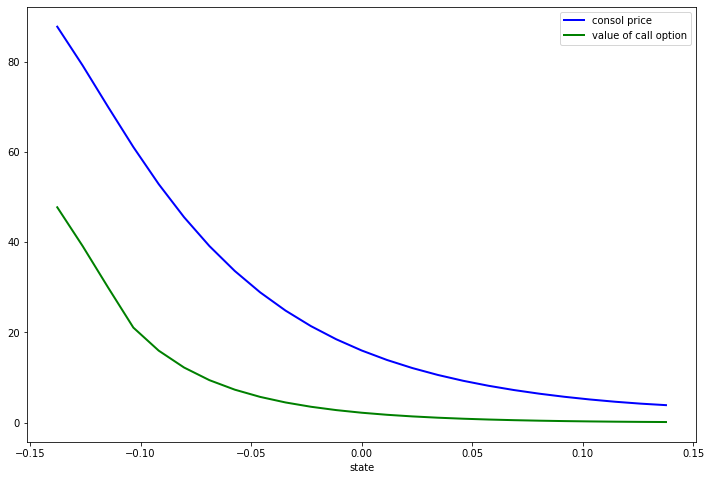In high values of the Markov growth state, the value of the option is close to zero.

This is despite the facts that the Markov chain is irreducible and that low states — where the consol prices are high — will be visited recurrently.

The reason for low valuations in high Markov growth states is that $\beta=0.9$, so future payoffs are discounted substantially.

### Risk-Free Rates¶

Let’s look at risk-free interest rates over different periods.

#### The One-period Risk-free Interest Rate¶

As before, the stochastic discount factor is $m_{t+1} = \beta g_{t+1}^{-\gamma}$.

It follows that the reciprocal $R_t^{-1}$ of the gross risk-free interest rate $R_t$ in state $x$ is

$${\mathbb E}_t m_{t+1} = \beta \sum_{y \in S} P(x, y) g(y)^{-\gamma}$$

We can write this as

$$m_1 = \beta M {\mathbb 1}$$

where the $i$-th element of $m_1$ is the reciprocal of the one-period gross risk-free interest rate in state $x_i$.

#### Other Terms¶

Let $m_j$ be an $n \times 1$ vector whose $i$ th component is the reciprocal of the $j$ -period gross risk-free interest rate in state $x_i$.

Then $m_1 = \beta M$, and $m_{j+1} = M m_j$ for $j \geq 1$.

## Exercises¶

### Exercise 1¶

In the lecture, we considered ex-dividend assets.

A cum-dividend asset is a claim to the stream $d_t, d_{t+1}, \ldots$.

Following (1), find the risk-neutral asset pricing equation for one unit of a cum-dividend asset.

With a constant, non-random dividend stream $d_t = d > 0$, what is the equilibrium price of a cum-dividend asset?

With a growing, non-random dividend process $d_t = g d_t$ where $0 < g \beta < 1$, what is the equilibrium price of a cum-dividend asset?

### Exercise 2¶

Consider the following primitives

In :
n = 5
P = 0.0125 * np.ones((n, n))
P += np.diag(0.95 - 0.0125 * np.ones(5))
# State values of the Markov chain
s = np.array([0.95, 0.975, 1.0, 1.025, 1.05])
γ = 2.0
β = 0.94


Let $g$ be defined by $g(x) = x$ (that is, $g$ is the identity map).

Compute the price of the Lucas tree.

Do the same for

• the price of the risk-free consol when $\zeta = 1$
• the call option on the consol when $\zeta = 1$ and $p_S = 150.0$

### Exercise 3¶

Let’s consider finite horizon call options, which are more common than the infinite horizon variety.

Finite horizon options obey functional equations closely related to (17).

A $k$ period option expires after $k$ periods.

If we view today as date zero, a $k$ period option gives the owner the right to exercise the option to purchase the risk-free consol at the strike price $p_S$ at dates $0, 1, \ldots , k-1$.

The option expires at time $k$.

Thus, for $k=1, 2, \ldots$, let $w(x, k)$ be the value of a $k$-period option.

It obeys

$$w(x, k) = \max \left\{ \beta \sum_{y \in S} P(x, y) g(y)^{-\gamma} w (y, k-1), \; p(x) - p_S \right\}$$

where $w(x, 0) = 0$ for all $x$.

We can express the preceding as the sequence of nonlinear vector equations

$$w_k = \max \{ \beta M w_{k-1}, \; p - p_S {\mathbb 1} \} \quad k =1, 2, \ldots \quad \text{with } w_0 = 0$$

Write a function that computes $w_k$ for any given $k$.

Compute the value of the option with k = 5 and k = 25 using parameter values as in Exercise 1.

Is one higher than the other? Can you give intuition?

## Solutions¶

### Exercise 1¶

For a cum-dividend asset, the basic risk-neutral asset pricing equation is

$$p_t = d_t + \beta {\mathbb E}_t [ p_{t+1} ]$$

With constant dividends, the equilibrium price is

$$p_t = \frac{1}{1-\beta} d_t$$

With a growing, non-random dividend process, the equilibrium price is

$$p_t = \frac{1}{1 - \beta g} d_t$$

### Exercise 2¶

First, let’s enter the parameters:

In :
n = 5
P = 0.0125 * np.ones((n, n))
P += np.diag(0.95 - 0.0125 * np.ones(5))
s = np.array([0.95, 0.975, 1.0, 1.025, 1.05])  # State values
mc = qe.MarkovChain(P, state_values=s)

γ = 2.0
β = 0.94
ζ = 1.0
p_s = 150.0


Next, we’ll create an instance of AssetPriceModel to feed into the functions

In :
apm = AssetPriceModel(β=β, mc=mc, γ=γ, g=lambda x: x)


Now we just need to call the relevant functions on the data:

In :
tree_price(apm)

Out:
array([29.47401578, 21.93570661, 17.57142236, 14.72515002, 12.72221763])
In :
consol_price(apm, ζ)

Out:
array([753.87100476, 242.55144082, 148.67554548, 109.25108965,
87.56860139])
In :
call_option(apm, ζ, p_s)

Out:
array([603.87100476, 176.8393343 , 108.67734499,  80.05179254,
64.30843748])

Let’s show the last two functions as a plot

In :
fig, ax = plt.subplots()
ax.plot(s, consol_price(apm, ζ), label='consol')
ax.plot(s, call_option(apm, ζ, p_s), label='call option')
ax.legend()
plt.show()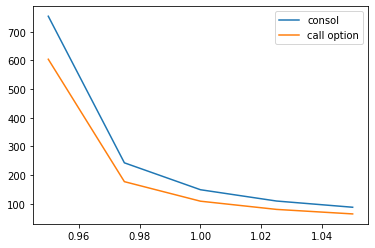### Exercise 3¶

Here’s a suitable function:

In :
def finite_horizon_call_option(ap, ζ, p_s, k):
"""
Computes k period option value.
"""
# Simplify names, set up matrices
β, γ, P, y = ap.β, ap.γ, ap.mc.P, ap.mc.state_values
M = P * ap.g(y)**(- γ)

# Make sure that a unique solution exists
ap.test_stability(M)

# Compute option price
p = consol_price(ap, ζ)
w = np.zeros(ap.n)
for i in range(k):
# Maximize across columns
w = np.maximum(β * M @ w, p - p_s)

return w


Now let’s compute the option values at k=5 and k=25

In :
fig, ax = plt.subplots()
for k in [5, 25]:
w = finite_horizon_call_option(apm, ζ, p_s, k)
ax.plot(s, w, label=rf'$k = {k}$')
ax.legend()
plt.show()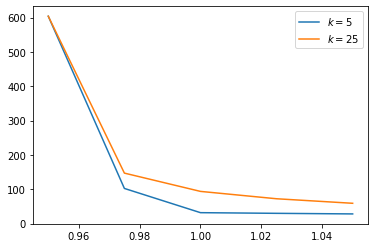Not surprisingly, the option has greater value with larger $k$.

This is because the owner has a longer time horizon over which he or she may exercise the option.

• Share page